# Functional Integration and Quantum Mechanics

### V5F5 - Summer semester 2020

Lecturers: F. de Vecchi and M. Gubinelli

Schedule: Tuesday 14.15-16.00 and Wednesday 8.30-10.00 (SemR 1.007)  Online until further notice

Please register in eCampus (link) if you would like to follow the course. Lectures will start the week of April 20th in an online format via Zoom, details on the connection will be shared via eCampus. Possibly the recording of the sessions will be made available offline via eCampus.

Exam: Oral exam (to be fixed)

### Topics of course

The course is an introduction to the study of Quantum Mechanical models via the connection with probability theory given by the Euclidean approach.

### Prerequisites

Basic knowledge of continuous time stocastic processes and some stochastic calculus, e.g. see my course "Foundations of Stochastic Analysis" from the WS19/20 (link). No previous knowledge of Quantum Mechanics will be assumed.

### References

• Strocchi, F. An Introduction to the Mathematical Structure of Quantum Mechanics: A Short Course for Mathematicians. 2 edition. New Jersey: World Scientific Publishing Company, 2008.

• Segal, E. E., and George Whitelaw Mackey. Mathematical Problems of Relativistic Physics. Providence, RI: American Mathematical Society, 1963.

• Meyer, Paul A. Quantum Probability for Probabilists. Springer, 1995.

•

### Lecture Notes

Lecture notes will be posted here as the course develops.

• Note 1 (version 1, 2020.5.5) [pdf]
•

### Course Journal

• Lecture 1 (21/4) Introduction, plan of the lectures. Introductio to QM: Stern-Gerlach experiment and quantum phenomena [script]
• Lecture 2 (22/4) Mathematical model of a physical system. Operational definition and properties of observables and states. Duality, algebraic structure. C*-algebras. Example of the description of a classical mechanical system. [script]
• Lecture 3 (28/4) C*-algebras, definition and first properties, spectrum of an element, spectral radius. multiplicative functionals. [script]
• Lecture 4 (29/4) Abelian Banach algebras, maximal ideals and multiplicative functionals, Gelfand transform, Gelfand-Naimark theorem on the structure of commutative C* algebras, functional calculus [script]
• Lecture 5 (5/5) Properties of positive elements, positive linear functionals (states) [script
• Lecture 6 (6/5) a remark on continuous functionals, GNS representation, representations of non-commutative C*-algebras, the non-commutative GN theorem. [script]
• Lecture 7 (12/5) irreducible representations and pure states, pure state of abelian algebras are multiplicative, probabilistic interpretation. [script]
• Lecture 8 (13/5) folium of a representation, Fell's theorem and physical interpretation, necessity of non-commutativity to describe quantum phenomenology, Heisenberg's indetermination principle and Planck's constant, quantum particle [script]
• Lecture 9 (19/5) (not recorded) Heisenberg indetermination principle, Schrödinger-Robertson inequality, canonical commutation relations (CCR), impossibility to realise the CCR with bounded operators, probabilistic phenomena in non-commutative algebras, construction of discrete systems of  complementary observables (to be continued)  [script]
• Lecture 10 (20/5) Conclusion of the construction of a system with two discrete complementary observables. Description of its pure states. An example of factorized system. Pauli matrices.  [script]
• Lecture 11 (26/5) Canonical commutation relations as limits of discrete ones.  Weyl algebra, Baker-Campbell-Hausdorff formula and relation with Heisenberg's commutation relations.  [script]
• Lecture 12 (27/5) Observables as *-homomorphism and unitary representations of R on Hilbert space. [script]
• Lecture 13 (2/6) Weyl algebra, Heisenberg group and von Neumann theorem on the uniqueness of irreducible regular representations of Weyl algebra in finite dimensions, Schrödinger representation, irreducibility [script]
• Lecture 14 (3/6) Determination of the vacuum in the Schrödinger representation. Gaussian representation of Weyl relations. Reducible representations. [script]
• Lecture 15 (9/6) An example of reducible representation, dynamics of a canonical pair  [script]
• Lecture 16 (10/6) Dynamics of a quantum system [script]
• Lecture 17 (16/6) Unitary representation of weakly continuous automorphism on an invariant state  [script]
• Lecture 18 (17/6) Semigroups and *-homomorphism, Bernstein theorem on totally monotone functions  [script]
• Lecture 19 (23/6) Link between unitary groups and self-adjoint semigroups. Energy operator.  [script]
• Lecture 20 (24/6) Positive energy condition. Link between unitary groups and self-adjoint semigroups continued. Ground states and Wightman functions. [script]
• Lecture 21 (30/6) Axioms for Wightman functions. [script]
• Lecture 22 (1/7) Axioms for Schwinger functions and the reconstruction theorem. [script]
• Lecture 23 (7/7) Completion of the proof of the reconstruction theorem. Reflection positivity for Gaussian processes. [script]
• Lecture 24 (8/7) Reflection positivity for the Ornstein-Uhlenbeck process. Explicit identification of the reconstruced Hilbert space as a probabilistic object, OU semigroup, generator, harmonic oscillator.  [script]
• Lecture 25 (14/7) More general setting for the reconstruction theorem. Nelson's positivity and stochastic processes. Reflection positivity for Gaussian processes  [script]
• Lecture 26 (15/7) Reflection positivity for Markov processes and construction or reflection positive processes via SDEs   [script]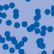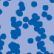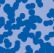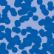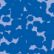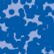## News

Florian Schweiger erhielt den Hausdorff-Gedächtnispreis 2021 der Fachgruppe Mathematik für die beste Dissertation. Er fertigte die Dissertation unter der Betreuung von Prof. Stefan Müller an. Unter anderen wurde Vanessa Ryborz mit einem Preis der Bonner Mathematischen Gesellschaft für ihre von Prof. Sergio Conti betreute Bachelorarbeit ausgezeichnet. (18.01.2022)

Prof. Dr. Sergio Albeverio has been elected into the Academia Europaea and the Accademia Nazionale dei Lincei (more; 02.12.2021).

Der SFB 1060 Die Mathematik der emergenten Effekte hat eine dritte Förderperiode erhalten. (26.11.20)

Prof. Dr. Andreas Eberle erhält den diesjährigen Lehrpreis der Universität Bonn. (22.07.2020)

Herr Dr. Richard Höfer erhielt den Hausdorff-Gedächtnispreis 2019 der Fachgruppe Mathematik für die beste Disseration. Betreut wurde die Arbeit von Prof. J. Velázquez (29.01.2020).

Contact

Managing Director: Prof. Dr. Massimiliano Gubinelli